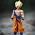» » » 10 Interesting Core Java Interview Coding Questions and Answers

# 10 Interesting Core Java Interview Coding Questions and Answers

#### 1. What is the output of following program?

1. package com.instanceofjava;
2.
3. public class B{
4.
5.   B b= new B();
6.
7.  public int show(){
8.       return (true ? null : 0);
9.  }
10.
11.  public static void main(String[] args)  {
12.
13.         B b= new B();
14.         b.show();
15.     }
16.
17. }

#### Exaplanation:

• Whenever we create the object of any class constructor will be called first and memory allocated for all non static variables
• Here  B b= new B(); variable is object and assigned to new object of same class
• B b= new B(); statement leads to recursive execution of constructor will create infinite objects so at run time an exception will be raised
• Exception in thread "main" java.lang.StackOverflowError
• The common cause for a stack overflow exception  is a bad recursive call. Typically this is caused when your recursive functions doesn't have the correct termination condition

#### 2. What is the output of following program?

1. package com.instanceofjava;
2.
3. public class A{

4.
5.  public static void show(){
6.
7.         System.out.println("Static method called");
8.  }
9.
10.  public static void main(String[] args)  {
11.
12.         A obj=null;
13.         obj.show();
14.
15.     }
16.
17. }

#### Exaplanation:

• We can call static methods using reference variable which is pointing to null because static methods are class level so we can either call using class name and reference variable which is pointing to null.

#### 3. What is the output of following program?

1. package com.instanceofjava;
2.
3. public class A{

4.
5.  static int a = 1111;
6.  static
7.  {
8.         a = a-- - --a;
9.  }
10.
11. {
12.         a = a++ + ++a;
13.  }
14.
15.  public static void main(String[] args)  {
16.
17.        System.out.println(a);
18.
19.     }
20.
21. }

#### 4. What is the output of following program?

1. package com.instanceofjava;
2.
3. public class A{

4.
5.  int GetValue()
6.  {
7.         return (true ? null : 0);
8.  }
9.
10.  public static void main(String[] args)  {
11.
12.    A obj= new A();

13.       obj.GetValue();
14.
15.     }
16.
17. }

#### 5. What is the output of following program?

1. package com.instanceofjava;
2.
3. public class A{

4.
5.  public static void main(String[] args)  {
6.
7.    Integer i1 = 128;
8.
9.    Integer i2 = 128;
10.
11.      System.out.println(i1 == i2);
12.
13.    Integer i3 = 127;
14.    Integer i4 = 127;
15.
16.       System.out.println(i3 == i4);
17.
18.     }
19.
20. }

#### 6. What is the output of following program?

1. package com.instanceofjava;
2. class A
3. {
4.
5. void method(int i)
6. {
7.
8.  }
9.
10.     }
11.
12.  class B extends A
13.  {
14.
15. @Override
16. void method(Integer i)
17.  {
18.
19.  }
20.

21. }

#### 7. Which line will throw compile time error? 8 or 9?

1. package com.instanceofjava;
2. class A
3. {
4.
5. public static void main(String [] args)
6. {
7.
8.   Integer i = new Integer(null);
9.   String s = new String(null);
10.
11.  }
12.
13. }

#### 8.What is the output of following program?

1. package com.instanceofjava;
2. class A
3. {
4.
5. public static void main(String [] args)
6. {
7.
8.   String s = "ONE"+3+2+"TWO"+"THREE"+5+4+"FOUR"+"FIVE"+5;
9.   System.out.println(s);
10.
11.  }
12.
13. }

#### 9.What is the output of following program?

1. package com.instanceofjava;
2. class A
3. {
4.
5. static int method1(int i)
6. {
7. return method2(i *= 11);
8. }
9.
10.  static int method2(int i)
11. {
12.   return method3(i /= 11);
13. }
14.
15.  static int method3(int i)
16. {
17.  return method4(i -= 11);
18. }
19.
20.  static int method4(int i)
21. {
22.   return i += 11;
23. }

24. public static void main(String [] args)
25. {
26.
27.   System.out.println(method1(11));
28.
29.  }
30.
31. }

#### 10.What is the output of following program?

1. package com.instanceofjava;
2. class A
3. {
4.
5. public static void main(String [] args)
6. {
7.
8.   System.out.println(null);

9.  }
10.
11. }

Explanation:

What happens When System.out.println(null)?

For more interview programs : Java Programs asked in interviews

#### 9 comments for 10 Interesting Core Java Interview Coding Questions and Answers

1.Hmm 3. What is the output of following program? rly? mb you try to use ide to see right answer?

1.nice suggestion. but we executed all programs before posting actually 3rd program out put will be 2 always even you change the value of a. its a logic based program.
a-- - --a=2 even you change the value of a. try it better anyway thanks for comment

2.Hello,
very Nice and Informative collection of Core Java Interview Coding Questions and Answers and thank you for sharing, I must admit few of these I never encountered.I also ask some of them at one of the am searching same java questions and answers Forum, I found your blog very Interesting . That really help me out in various queries of core java.
Thanks

3.How it will going to recursive
public class B
{

B b= new B();

public void show()
{

}

public static void main(String[] args)
{

B b1= new B();
b1.show();
System.out.println("done");
}

}

4.Thankyou ... Was useful

5.useful....thanks

6.useful thanks

7.class A{

{
System.out.print("compiles without error how?")
}
}

8.Really good questions.Thanks for sharing.